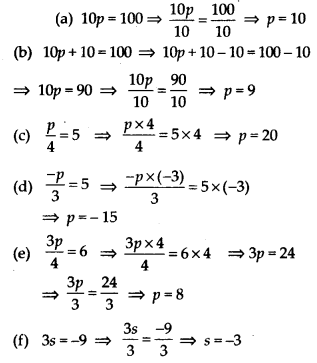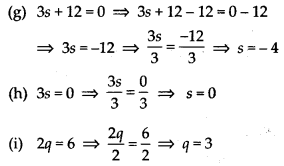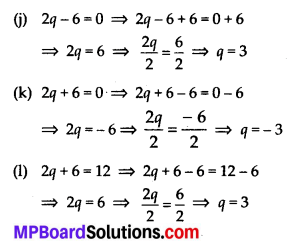# MP Board Class 7th Maths Solutions Chapter 4 Simple Equations Ex 4.2

## MP Board Class 7th Maths Solutions Chapter 4 Simple Equations Ex 4.2

Question 1.
Give first the step you will use to separate the variable and then solve the equation:
(a) x – 1 = 0
(b) x + 1 = 0
(c) x – 1 = 5
(d) x + 6 = 2
(e) y – 4 = -7
(f) y – 4 = 4
(g) y + 4 = 4
(h) y + 4 = -4
Solution:
On adding 1 to both sides of the given equation, we obtain
x – 1 + 1 = 0 + 1 ⇒ x = 1

(b) x + 1 = 0
On subtracting 1 from both sides of the given equation, we obtain
x + 1 – 1 = 0 – 1 ⇒ x = -1

(c) x – 1 = 5
On adding 1 to both sides of the given equation, we obtain
x – 1 + 1 = 5 + 1 ⇒ x = 6

(d) x + 6 = 2
On subtracting 6 from both sides of the given equation, we obtain
x + 6 – 6 = 2 – 6 ⇒ x = -4

(e) y – 4 = – 7
On adding 4 to both sides of the given equation, we obtain
y – 4 + 4 = -7 + 4 ⇒ y = -3

(f) y – 4 = 4
On adding 4 to both sides of the given equation, we obtain
y – 4 + 4 = 4 + 4 ⇒ y = 8

(g) y + 4 = 4
On subtracting 4 from both sides of the given equation, we obtain
y + 4 – 4 = 4 – 4 ⇒ y = 0

(h) y + 4 = -4
On subtracting 4 from both sides of the given equation, we obtain
y + 4 – 4 = – 4 – 4 ⇒ y = -8Question 2.
Give first the step you will use to separate the variable and then solve the equation:
(a) 3l = 42
(b) $$\frac{b}{2}$$ = 6
(c) $$\frac{p}{7}$$ = 4
(d) 4x = 25
(e) 8y = 36
(f) $$\frac{z}{3}=\frac{5}{4}$$
(g) $$\frac{a}{5}=\frac{7}{15}$$
(h) 20t = -10
Solution:
(a) 3l = 42
On dividing both sides of the given equation by 3, we obtain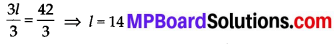(b) $$\frac{b}{2}$$ = 6
On multiplying both sides of the given equation by 2, we obtain(c) $$\frac{p}{7}$$ = 4
On multiplying both sides of the given equation by 7, we obtain(d) 4x = 25
On dividing both sides of the given equation by 4, we obtain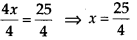(e) 8y = 36
On dividing both sides of the given equation by 8, we obtain(f) $$\frac{z}{3}=\frac{5}{4}$$
On multiplying both sides of the given equation by 3, we obtain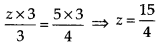(g) $$\frac{a}{5}=\frac{7}{15}$$
On multiplying both sides of the given equation by 5, we obtain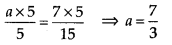(h) 20t = -10
On dividing both sides of the given equation by 20, we obtain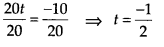Question 3.
Give the steps you will use to separate the variable and then solve the equation:
(a) 3n – 2 = 46
(b) 5m + 7 = 17
(c) $$\frac{20 p}{3}$$ = 40
(d) $$\frac{3 p}{10}$$ = 6
Solution:
(a) 3n – 2 = 46
On adding 2 to both sides, we obtain
3n – 2 + 2 = 46 + 2 ⇒ 3n = 48
On dividing both sides of the given equation by 3, we obtain(b) 5m + 7 = 17
On subtracting 7 from both sides, we obtain
5m + 7 – 7 = 17 – 7 ⇒ 5m = 10
On dividing both sides of the given equation by 5, we obtain(c) $$\frac{20 p}{3}$$ = 40
On multiplying both sides of the given equation by 3, we obtain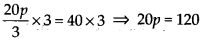On dividing both sides of the given equation by 20, we obtain(d) $$\frac{3 p}{10}$$ = 6
On multiplying both sides of the given equation by 10, we obtain 3pxl0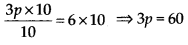On dividing both sides of the given equation by 3, we obtainQuestion 4.
Solve the following equations:
(a) 10p = 100
(b) 10p + 10 = 100
(c) $$\frac{p}{4}$$ = 5
(d) $$\frac{-p}{3}$$ = 5
(e) $$\frac{39}{4}$$ = 6
(f) 3s = -9
(g) 3s + 12 = 0
(h) 3s = 0
(i) 2q = 6
(j) 2q – 6 = 0
(k) 2q + 6 = 0
(l) 2q + 6 = 12
Solution: